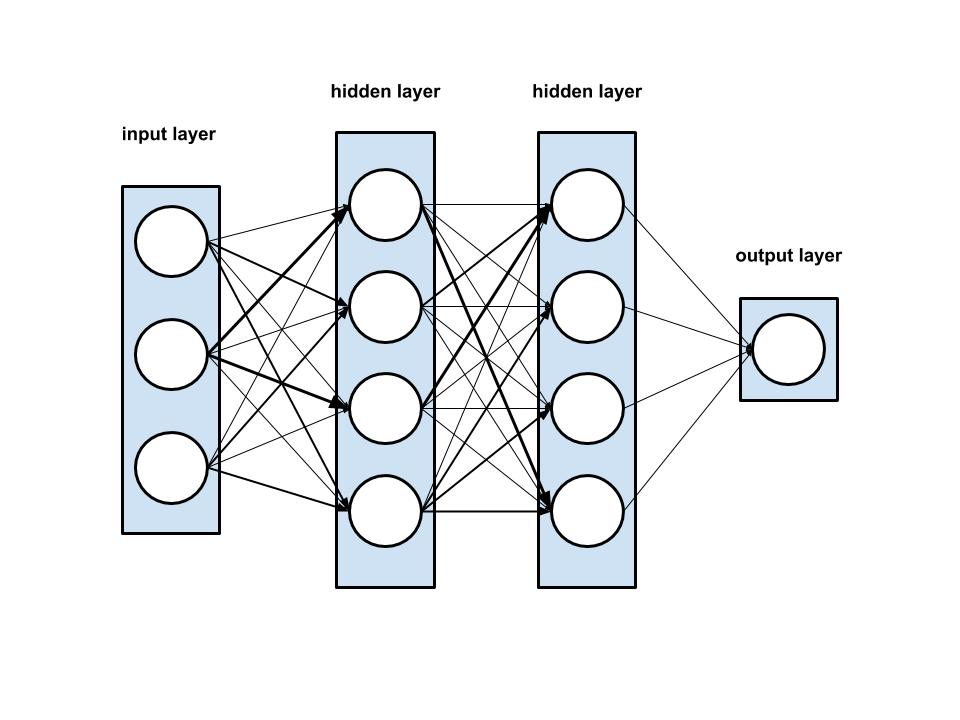# Understanding the Basics of Neural Networks

For some years now, machine learning has been one of the most celebrated and explored areas of technological development. In particular, deep learning and neural networks have fascinating potential. To illustrate how neural networks function and to demonstrate their success, we'll explain a naive implementation of a simple neural network using Java.

## An Introduction to Deep Learning and Neural Networks

The goal of deep learning is, in an abstract way, to emulate some basic principles of the biology that makes up the human brain. Deep learning models are made of neural networks composed of nodes (which may be compared to the brain's neurons, which are simple processing structures) and the connections between nodes (which may be compared to the brain's synapses). While each neuron in the human brain can have about a trillion connections, artificial networks usually work with smaller quantities of nodes and connections. Even so, they have an impressive ability to process many different problems that range from natural language approximation to image recognition.

Regression functions are at the core of neural networks. They are the math that helps neural networks "learn" through data training. For example, regression functions can help the network recognize a dog in a picture. These mathematical functions train the network to weight its parameters and move forward to a more precise classification of its training data until it is adjusted to be exposed to new data.

While anyone interested in deep learning needs to know how these mathematical functions allow neural networks to classify and forecast data trends, the functions are complex and can be a barrier those exploring the field. Today, we'll examine a naive implementation of a simple neural network using Java to show how NNs work. Although neural networks are usually implemented with the mathematical model using matrices operations, we'll try to explain their basic functionality by describing their conceptual representations.

## The Neuron

The first class in the neural network model is the node, and it is analogous to a neuron. There are three components that constitute a node: the weights, the activation function, and the bias. Weights allow values to be adjusted through training sets so the neural network can learn and find patterns in new and unknown data.From: Wikipedia

The activation function defines the output of the node given the inputs it receives, and the bias serves as a trainable constant value in the function. The image above shows a representation of the inputs through the activation function, where, if the inputs reach a certain threshold, they will be passed along to some extent to other nodes in the network.

## The Layer

The second class in the neural network model is the layer. Each layer in a neural network is comprised of a set of nodes, making it a multi-layer neural network. The layers in a neural network serve three different purposes. First, there is the input layer, which is responsible for inputting data into the NN. Next, there is the output layer, which gives the results of data processed through the NN. Finally, there are hidden layers, which reside between the input and output layers. There can be any number of hidden layers in the NN, and adjusting the NN to include the optimal number of hidden layers and nodes is crucial when working with more complex data.

## The Network

As depicted below, the network itself is constituted of methods for training and testing:## Training Our Neural Network

Without training, our neural network is as good as a random guess. Our test network has thousands of weights and biases that can be adjusted to improve its accuracy. By changing a single weight, the network can perform better or worse against a training data set. If we repeat this process for several iterations and keep only the improvements, the network will gradually improve as a whole.

With this basic understanding of the components of this neural network, we can run some tests using the famous Iris dataset. The Iris dataset is comprised of three different classes, each of which refers to a type of Iris plant. Each line in the dataset contains an instance of a plant, with values corresponding to the characteristics of the specific plant. The test method is as follows: one test run yielded 99.4% accuracy for the training data and 95.1% for the test data set. (If you want to learn more, you can find the repository with the complete project here.)

## Conclusion

With the implementation of basic Neural Network concepts, it’s already possible to get good results from pattern recognition challenges, like the Iris data set, with great efficiency. Even though there is a lot of room for improvement within the field of deep learning, results like these make it clear why neural networks have garnered great attention within the broader field of machine learning and why we at Avenue Code are excited to continue pioneering machine learning possibilities.

(This article was co-authored with Luis Felipe Talvik, Software Engineer at Avenue Code.)

# Anderson Alves de Sá

Anderson Alves de Sa is a Java Software Engineer at Avenue Code with experience in system analysis, development, integration, testing, and deployment.